The machine learning models have started penetrating into critical areas like health care, justice systems, and financial industry. Thus to figure out how the models make the decisions and make sure the decisioning process is aligned with the ethnic requirements or legal regulations becomes a necessity.

Meanwhile, the rapid growth of deep learning models pushes the requirement of interpreting complicated models further. People are eager to apply the power of AI fully on key aspects of everyday life. However, it is hard to do so without enough trust in the models or an efficient procedure to explain unintended behavior, especially considering that the deep neural networks are born as black-boxes.

Think of the following cases:

1. The financial industry is highly regulated and loan issuers are required by law to make fair decisions and explain their credit models to provide reasons whenever they decide to decline loan application.
2. Medical diagnosis model is responsible for human life. How can we be confident enough to treat a patient as instructed by a black-box model?
3. When using a criminal decision model to predict the risk of recidivism at the court, we have to make sure the model behaves in an equitable, honest and nondiscriminatory manner.
4. If a self-driving car suddenly acts abnormally and we cannot explain why, are we gonna be comfortable enough to use the technique in real traffic in large scale?

At Affirm, we are issuing tens of thousands of installment loans every day and our underwriting model has to provide declination reasons when the model rejects one’s loan application. That’s one of the many motivations for me to dig deeper and write this post. Model interpretability is a big field in machine learning. This review is never met to exhaust every study, but to serve as a starting point.

# Interpretable Models#

Lipton (2017) summarized the properties of an interpretable model in a theoretical review paper, “The mythos of model interpretability”: A human can repeat (“simulatability”) the computation process with a full understanding of the algorithm (“algorithmic transparency”) and every individual part of the model owns an intuitive explanation (“decomposability”).

Many classic models have relatively simpler formation and naturally, come with a model-specific interpretation method. Meanwhile, new tools are being developed to help create better interpretable models (Been, Khanna, & Koyejo, 2016; Lakkaraju, Bach & Leskovec, 2016).

## Regression#

A general form of a linear regression model is:

$$y = w_0 + w_1 x_1 + w_2 x_2 + … + w_n x_n$$

The coefficients describe the change of the response triggered by one unit increase of the independent variables. The coefficients are not comparable directly unless the features have been standardized (check sklearn.preprocessing.StandardScalar and RobustScaler), since one unit of different features can refer to very different things. Without standardization, the product $w_i \dot x_i$ can be used to quantify one feature’s contribution to the response.

## Naive Bayes#

Naive Bayes is named as “Naive” because it works on a very simplified assumption that features are independent of each other and each contributes to the output independently.

Given a feature vector $\mathbf{x} = [x_1, x_2, \dots, x_n]$ and a class label $c \in \{1, 2, \dots, C\}$, the probability of this data point belonging to this class is:

\begin{aligned} p(c | x_1, x_2, \dots, x_n) &\propto p(c, x_1, x_2, \dots, x_n)\\ &\propto p(c) p(x_1 | c) p(x_2 | c) \dots p(x_n | c)\\ &\propto p(c) \prod_{i=1}^n p(x_i | c). \end{aligned}

The Naive Bayes classifier is then defined as:

$$\hat{y} = \arg\max_{c \in 1, \dots, C} p(c) \prod_{i=1}^n p(x_i | c)$$

Because the model has learned the prior $p(x_i \vert c)$ during the training, the contribution of an individual feature value can be easily measured by the posterior, $p(c \vert x_i) = p(c)p(x_i \vert c) / p(x_i)$.

## Decision Tree/Decision Lists#

Decision lists are a set of boolean functions, usually constructed by the syntax like if... then... else.... The if-condition contains a function involving one or multiple features and a boolean output. Decision lists are born with good interpretability and can be visualized in a tree structure. Many research on decision lists is driven by medical applications, where the interpretability is almost as crucial as the model itself.

A few types of decision lists are briefly described below:

• Falling Rule Lists (FRL) (Wang and Rudin, 2015) has fully enforced monotonicity on feature values. One key point, for example in the binary classification context, is that the probability of prediction $Y=1$ associated with each rule decreases as one moves down the decision lists.
• Bayesian Rule List (BRL) (Letham et al., 2015) is a generative model that yields a posterior distribution over possible decision lists.
• Interpretable Decision Sets (IDS) (Lakkaraju, Bach & Leskovec, 2016) is a prediction framework to create a set of classification rules. The learning is optimized for both accuracy and interpretability simultaneously. IDS is closely related to the BETA method I’m gonna describe later for interpreting black-box models.

## Random Forests#

Weirdly enough, many people believe that the Random Forests model is a black box, which is not true. Considering that the output of random forests is the majority vote by a large number of independent decision trees and each tree is naturally interpretable.

It is not very hard to gauge the influence of individual features if we look into a single tree at a time. The global feature importance of random forests can be quantified by the total decrease in node impurity averaged over all trees of the ensemble (“mean decrease impurity”).

For one instance, because the decision paths in all the trees are well tracked, we can use the difference between the mean value of data points in a parent node between that of a child node to approximate the contribution of this split. Read more in this series of blog posts: Interpreting Random Forests.

# Interpreting Black-Box Models#

A lot of models are not designed to be interpretable. Approaches to explaining a black-box model aim to extract information from the trained model to justify its prediction outcome, without knowing how the model works in details. To keep the interpretation process independent from the model implementation is good for real-world applications: Even when the base model is being constantly upgraded and refined, the interpretation engine built on top would not worry about the changes.

Without the concern of keeping the model transparent and interpretable, we can endow the model with greater power of expressivity by adding more parameters and nonlinearity computation. That’s how deep neural networks become successful in tasks involving rich inputs.

There is no hard requirement on how the explanation should be presented, but the primary goal is mainly to answer: Can I trust this model? When we rely on the model to make a critical or life-and-death decision, we have to make sure the model is trustworthy ahead of time.

The interpretation framework should balance between two goals:

• Fidelity: the prediction produced by an explanation should agree with the original model as much as possible.
• Interpretability: the explanation should be simple enough to be human-understandable.

Side Notes: The next three methods are designed for local interpretation.

## Prediction Decomposition#

Robnik-Sikonja and Kononenko (2008) proposed to explain the model prediction for one instance by measuring the difference between the original prediction and the one made with omitting a set of features.

Let’s say we need to generate an explanation for a classification model $f: \mathbf{X} \rightarrow \mathbf{Y}$. Given a data point $x \in X$ which consists of $a$ individual values of attribute $A_i$, $i = 1, \dots, a$, and is labeled with class $y \in Y$. The prediction difference is quantified by computing the difference between the model predicted probabilities with or without knowing $A_i$:

$$\text{probDiff}_i (y | x) = p(y| x) - p(y | x \backslash A_i)$$

(The paper also discussed on using the odds ratio or the entropy-based information metric to quantify the prediction difference.)

Problem: If the target model outputs a probability, then great, getting $p(y \vert x)$ is straightforward. Otherwise, the model prediction has to run through an appropriate post-modeling calibration to translate the prediction score into probabilities. This calibration layer is another piece of complication.

Another problem: If we generate $x \backslash A_i$ by replacing $A_i$ with a missing value (like None, NaN, etc.), we have to rely on the model’s internal mechanism for missing value imputation. A model which replaces these missing cases with the median should have output very different from a model which imputes a special placeholder. One solution as presented in the paper is to replace $A_i$ with all possible values of this feature and then sum up the prediction weighted by how likely each value shows in the data:

\begin{aligned} p(y \vert x \backslash A_i) &= \sum_{s=1}^{m_i} p(A_i=a_s \vert x \backslash A_i) p(y \vert x \leftarrow A_i=a_s) \\ &\approx \sum_{s=1}^{m_i} p(A_i=a_s) p(y \vert x \leftarrow A_i=a_s) \end{aligned}

Where $p(y \vert x \leftarrow A_i=a_s)$ is the probability of getting label $y$ if we replace the feature $A_i$ with value $a_s$ in the feature vector of $x$. There are $m_i$ unique values of $A_i$ in the training set.

With the help of the measures of prediction difference when omitting known features, we can decompose the impact of each individual feature on the prediction.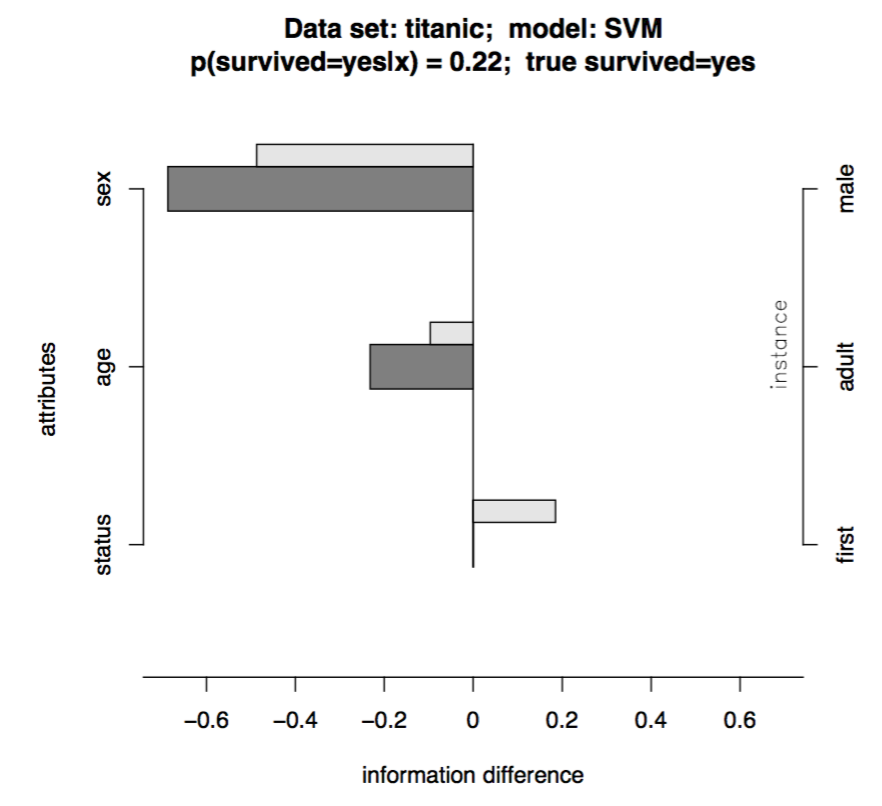This method (Baehrens, et al. 2010) is able to explain the local decision taken by arbitrary nonlinear classification algorithms, using the local gradients that characterize how a data point has to be moved to change its predicted label.

Let’s say, we have a Bayes Classifier which is trained on the data set $X$ and outputs probabilities over the class labels $Y$, $p(Y=y \vert X=x)$. And one class label $y$ is drawn from the class label pool, $\{1, 2, \dots, C\}$. This Bayes classifier is constructed as:

$$f^{*}(x) = \arg \min_{c \in \{1, \dots, C\}} p(Y \neq c \vert X = x)$$

The local explanation vector is defined as the derivative of the probability prediction function at the test point $x = x_0$. A large entry in this vector highlights a feature with a big influence on the model decision; A positive sign indicates that increasing the feature would lower the probability of $x_0$ assigned to $f^{*}(x_0)$.

However, this approach requires the model output to be a probability (similar to the “Prediction Decomposition” method above). What if the original model (labelled as $f$) is not calibrated to yield probabilities? As suggested by the paper, we can approximate $f$ by another classifier in a form that resembles the Bayes classifier $f^{*}$:

(1) Apply Parzen window to the training data to estimate the weighted class densities:

$$\hat{p}_{\sigma}(x, y=c) = \frac{1}{n} \sum_{i \in I_c} k_{\sigma} (x - x_i)$$

Where $I_c$ is the index set containing the indices of data points assigned to class $c$ by the model $f$, $I_c = \{i \vert f(x_i) = c\}$. $k_{\sigma}$ is a kernel function. Gaussian kernel is a popular one among many candidates.

(2) Then, apply the Bayes' rule to approximate the probability $p(Y=c \vert X=x)$ for all classes:

\begin{aligned} \hat{p}_{\sigma}(y=c | x) &= \frac{\hat{p}_{\sigma}(x, y=c)}{\hat{p}_{\sigma}(x, y=c) + \hat{p}_{\sigma}(x, y \neq c)} \\ &\approx \frac{\sum_{i \in I_c} k_{\sigma} (x - x_i)}{\sum_i k_{\sigma} (x - x_i)} \end{aligned}

(3) The final estimated Bayes classifier takes the form:

$$\hat{f}_{\sigma} = \arg\min_{c \in \{1, \dots, C\}} \hat{p}_{\sigma}(y \neq c \vert x)$$

Noted that we can generate the labeled data with the original model $f$, as much as we want, not restricted by the size of the training data. The hyperparameter $\sigma$ is selected to optimize the chances of $\hat{f}_{\sigma}(x) = f(x)$ to achieve high fidelity.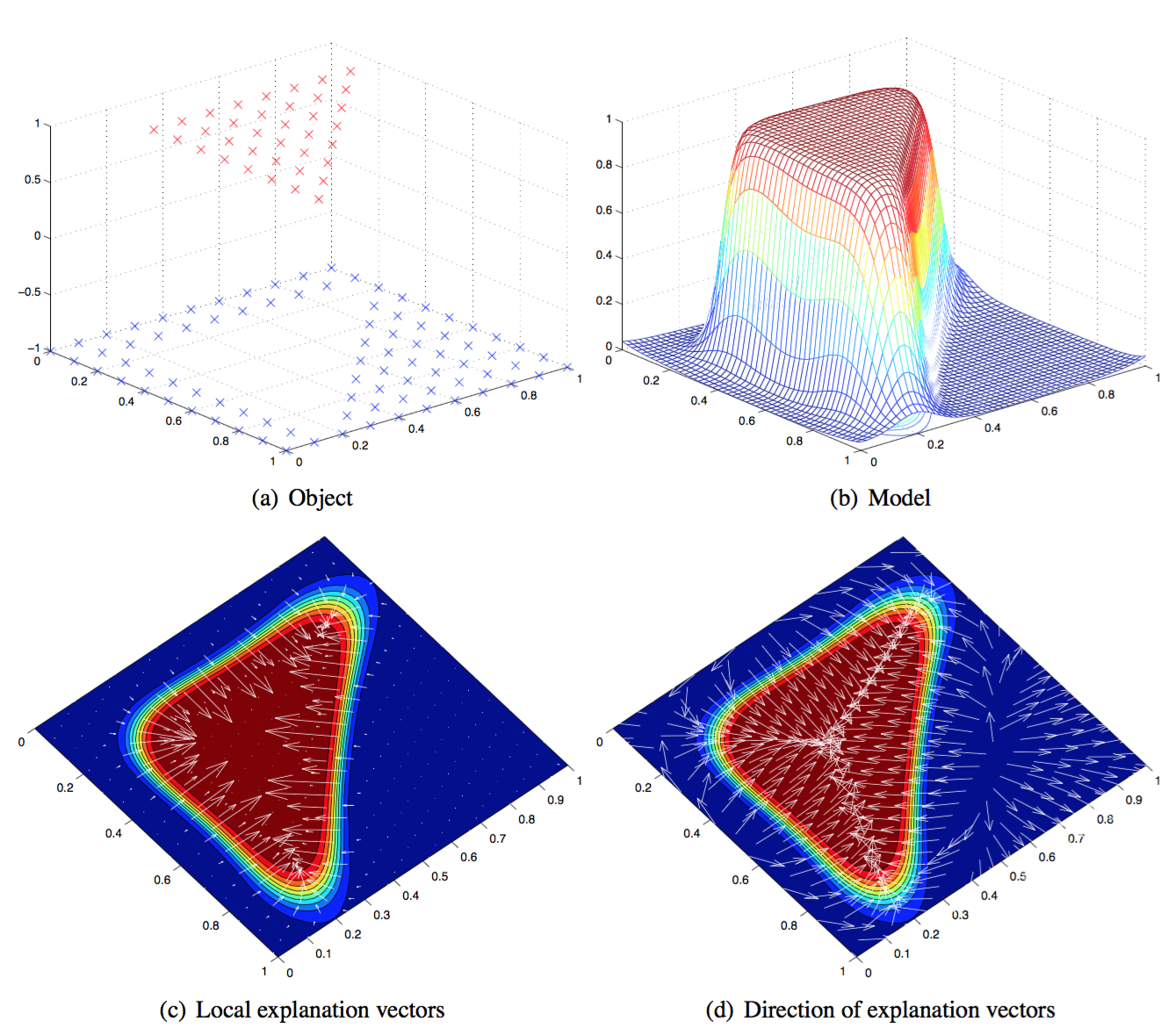Side notes: As you can see both the methods above require the model prediction to be a probability. Calibration of the model output adds another layer of complication.

## LIME (Local Interpretable Model-Agnostic Explanations)#

LIME, short for local interpretable model-agnostic explanation, can approximate a black-box model locally in the neighborhood of the prediction we are interested (Ribeiro, Singh, & Guestrin, 2016).

Same as above, let us label the black-box model as $f$. LIME presents the following steps:

(1) Convert the dataset into interpretable data representation: $x \Rightarrow x_b$.

• Text classifier: a binary vector indicating the presence or absence of a word
• Image classifier: a binary vector indicating the presence or absence of a contiguous patch of similar pixels (super-pixel).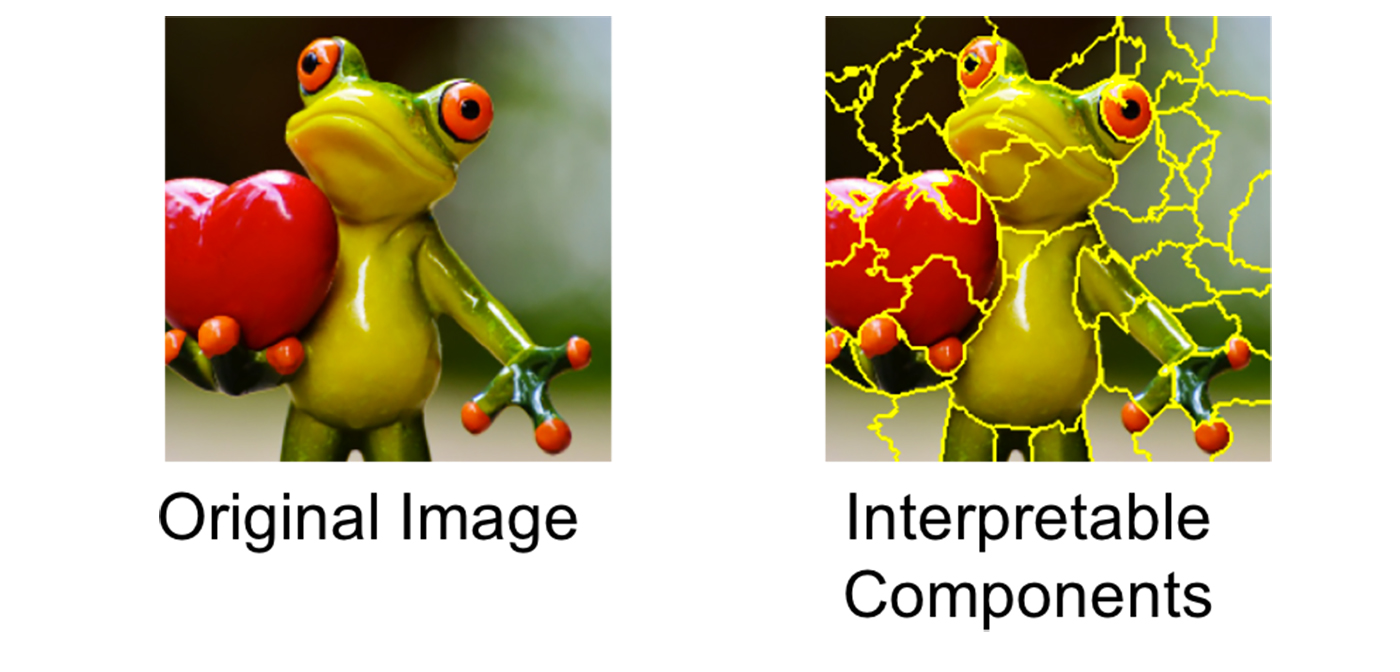(2) Given a prediction $f(x)$ with the corresponding interpretable data representation $x_b$, let us sample instances around $x_b$ by drawing nonzero elements of $x_b$ uniformly at random where the number of such draws is also uniformly sampled. This process generates a perturbed sample $z_b$ which contains a fraction of nonzero elements of $x_b$.

Then we recover $z_b$ back into the original input $z$ and get a prediction score $f(z)$ by the target model.

Use many such sampled data points $z_b \in \mathcal{Z}_b$ and their model predictions, we can learn an explanation model (such as in a form as simple as a regression) with local fidelity. The sampled data points are weighted differently based on how close they are to $x_b$. The paper used a lasso regression with preprocessing to select top $k$ most significant features beforehand, named “K-LASSO”.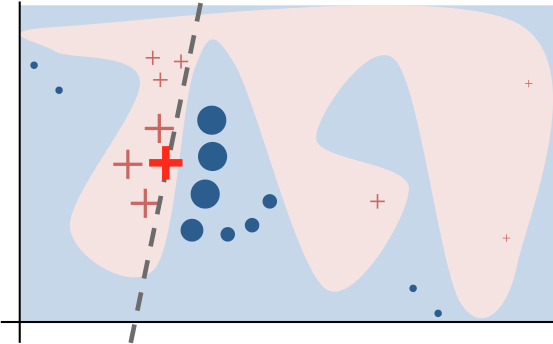Examining whether the explanation makes sense can directly decide whether the model is trustworthy because sometimes the model can pick up spurious correlation or generalization. One interesting example in the paper is to apply LIME on an SVM text classifier for differentiating “Christianity” from “Atheism”. The model achieved a pretty good accuracy (94% on held-out testing set!), but the LIME explanation demonstrated that decisions were made by very arbitrary reasons, such as counting the words “re”, “posting” and “host” which have no connection with neither “Christianity” nor “Atheism” directly. After such a diagnosis, we learned that even the model gives us a nice accuracy, it cannot be trusted. It also shed lights on ways to improve the model, such as better preprocessing on the text.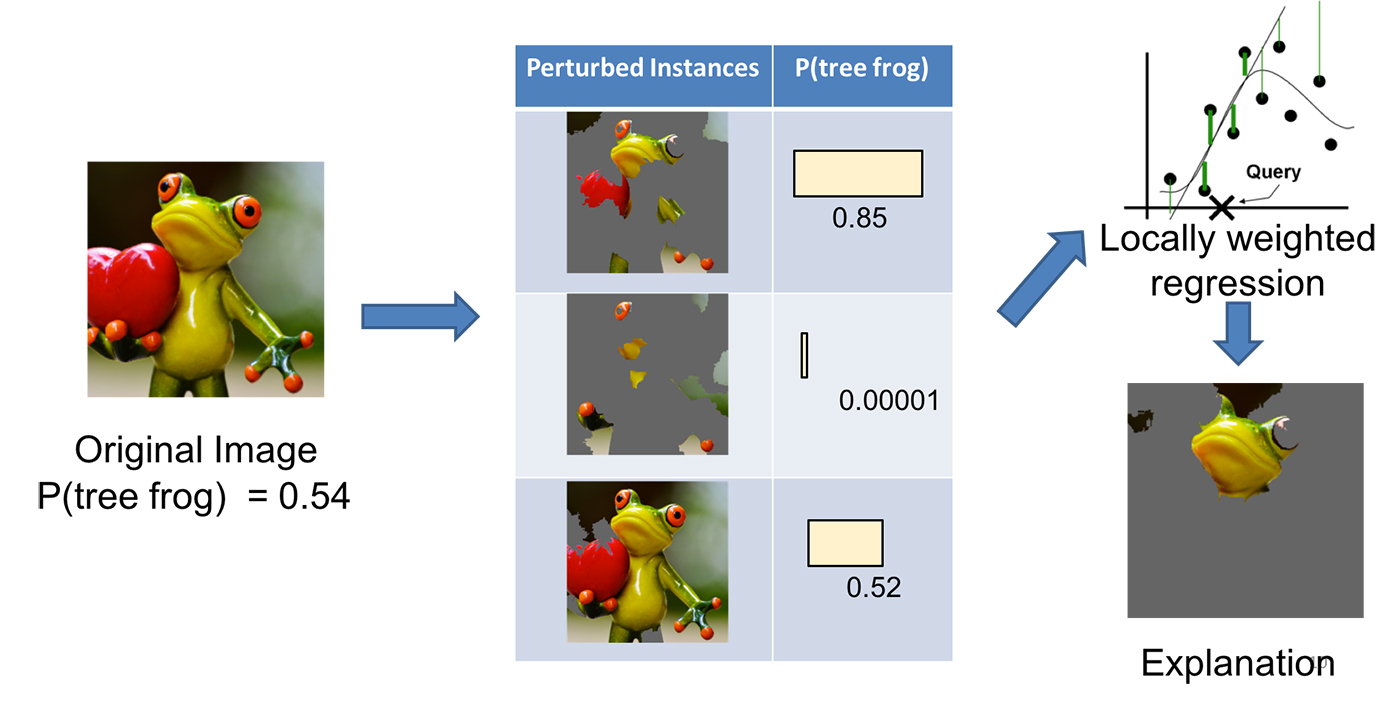For more detailed non-paper explanation, please read this blog post by the author. A very nice read.

Side Notes: Interpreting a model locally is supposed to be easier than interpreting the model globally, but harder to maintain (thinking about the curse of dimensionality). Methods described below aim to explain the behavior of a model as a whole. However, the global approach is unable to capture the fine-grained interpretation, such as a feature might be important in this region but not at all in another.

## Feature Selection#

Essentially all the classic feature selection methods (Yang and Pedersen, 1997; Guyon and Elisseeff, 2003) can be considered as ways to explain a model globally. Feature selection methods decompose the contribution of multiple features so that we can explain the overall model output by individual feature impact.

There are a ton of resources on feature selection so I would skip the topic in this post.

## BETA (Black Box Explanation through Transparent Approximations)#

BETA, short for black box explanation through transparent approximations, is closely connected to Interpretable Decision Sets (Lakkaraju, Bach & Leskovec, 2016). BETA learns a compact two-level decision set in which each rule explains part of the model behavior unambiguously.

The authors proposed an novel objective function so that the learning process is optimized for high fidelity (high agreement between explanation and the model), low unambiguity (little overlaps between decision rules in the explanation), and high interpretability (the explanation decision set is lightweight and small). These aspects are combined into one objection function to optimize for.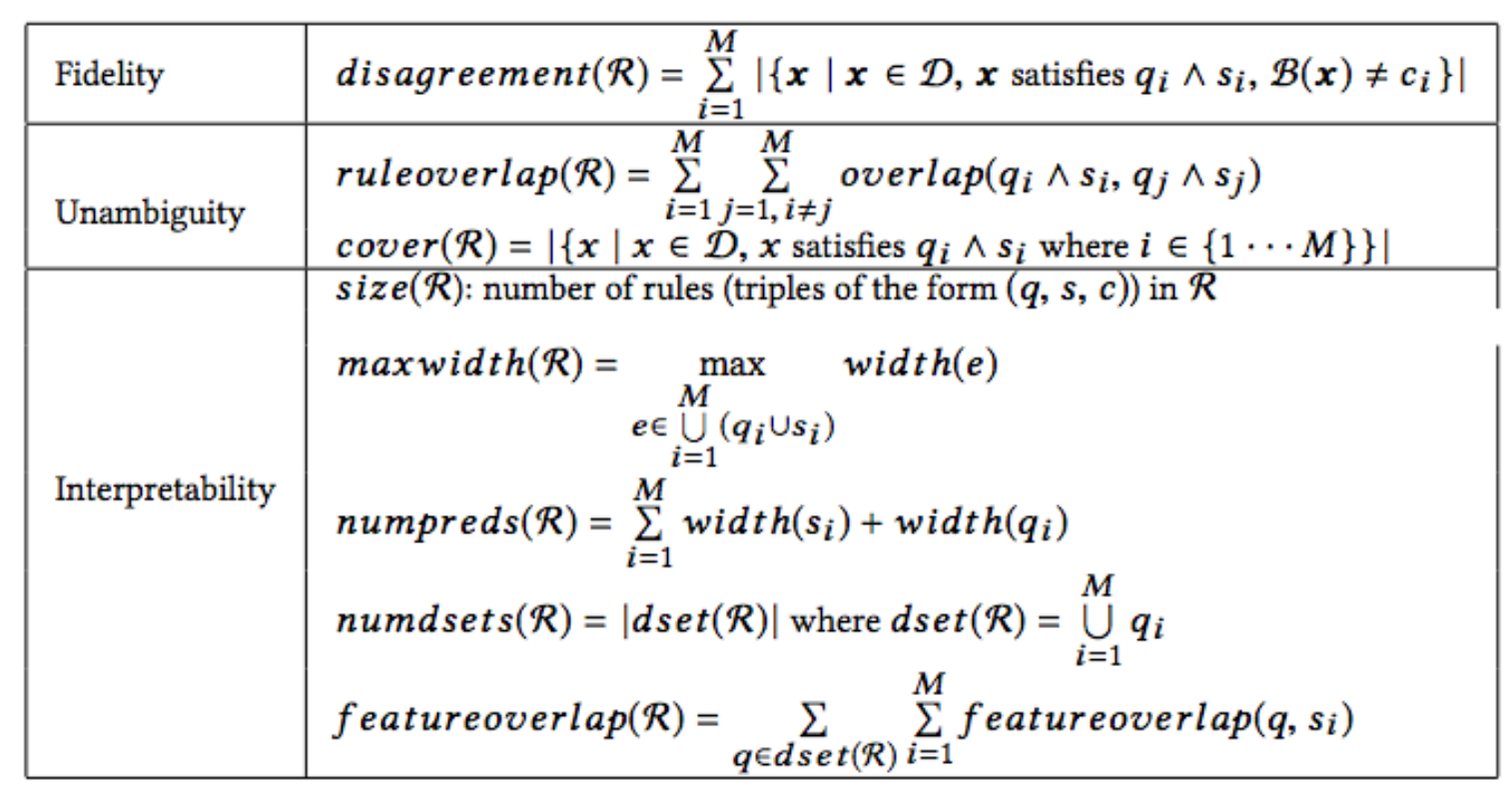# Explainable Artificial Intelligence#

I borrow the name of this section from the DARPA project “Explainable Artificial Intelligence”. This Explainable AI (XAI) program aims to develop more interpretable models and to enable human to understand, appropriately trust, and effectively manage the emerging generation of artificially intelligent techniques.

With the progress of the deep learning applications, people start worrying about that we may never know even if the model goes bad. The complicated structure, the large number of learnable parameters, the nonlinear mathematical operations and some intriguing properties (Szegedy et al., 2014) lead to the un-interpretability of deep neural networks, creating a true black-box. Although the power of deep learning is originated from this complexity — more flexible to capture rich and intricate patterns in the real-world data.

Studies on adversarial examples (OpenAI Blog: Robust Adversarial Examples, Attacking Machine Learning with Adversarial Examples, Goodfellow, Shlens & Szegedy, 2015; Nguyen, Yosinski, & Clune, 2015) raise the alarm on the robustness and safety of AI applications. Sometimes the models could show unintended, unexpected and unpredictable behavior and we have no fast/good strategy to tell why.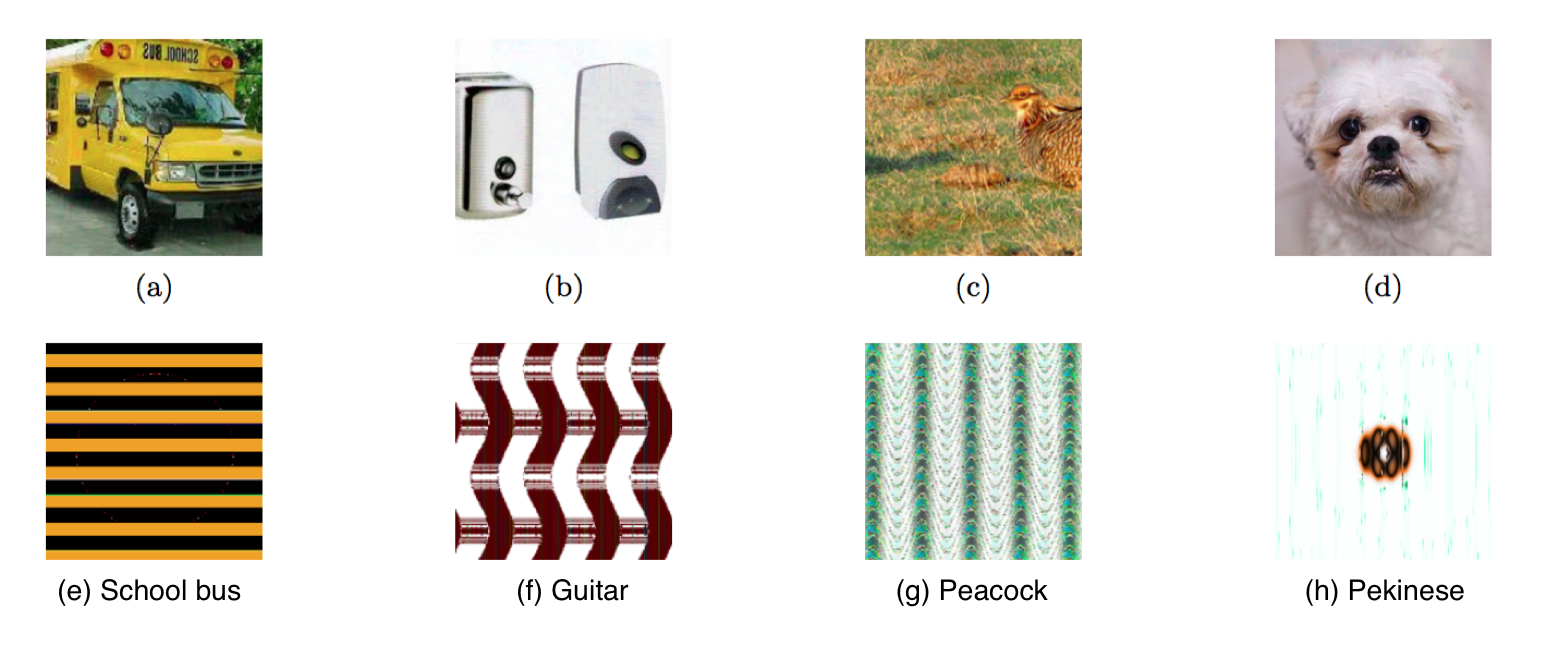Nvidia recently developed a method to visualize the most important pixel points in their self-driving cars' decisioning process. The visualization provides insights on how AI thinks and what the system relies on while operating the car. If what the AI believes to be important agrees with how human make similar decisions, we can naturally gain more confidence in the black-box model.

Many exciting news and findings are happening in this evolving field every day. Hope my post can give you some pointers and encourage you to investigate more into this topic :)

Cited as:

@article{weng2017gan,
title   = "How to Explain the Prediction of a Machine Learning Model?",
author  = "Weng, Lilian",
journal = "lilianweng.github.io",
year    = "2017",
url     = "https://lilianweng.github.io/posts/2017-08-01-interpretation/"
}


 Zachary C. Lipton. “The mythos of model interpretability." arXiv preprint arXiv:1606.03490 (2016).

 Been Kim, Rajiv Khanna, and Oluwasanmi O. Koyejo. “Examples are not enough, learn to criticize! criticism for interpretability.” Advances in Neural Information Processing Systems. 2016.

 Himabindu Lakkaraju, Stephen H. Bach, and Jure Leskovec. “Interpretable decision sets: A joint framework for description and prediction." Proc. 22nd ACM SIGKDD Intl. Conf. on Knowledge Discovery and Data Mining. ACM, 2016.

 Robnik-Šikonja, Marko, and Igor Kononenko. “Explaining classifications for individual instances." IEEE Transactions on Knowledge and Data Engineering 20.5 (2008): 589-600.

 Baehrens, David, et al. “How to explain individual classification decisions." Journal of Machine Learning Research 11.Jun (2010): 1803-1831.

 Marco Tulio Ribeiro, Sameer Singh, and Carlos Guestrin. “Why should I trust you?: Explaining the predictions of any classifier." Proc. 22nd ACM SIGKDD Intl. Conf. on Knowledge Discovery and Data Mining. ACM, 2016.

 Yiming Yang, and Jan O. Pedersen. “A comparative study on feature selection in text categorization." Intl. Conf. on Machine Learning. Vol. 97. 1997.

 Isabelle Guyon, and André Elisseeff. “An introduction to variable and feature selection." Journal of Machine Learning Research 3.Mar (2003): 1157-1182.

 Ian J. Goodfellow, Jonathon Shlens, and Christian Szegedy. “Explaining and harnessing adversarial examples." ICLR 2015.

 Christian Szegedy, Wojciech Zaremba, Ilya Sutskever, Joan Bruna, Dumitru Erhan, Ian Goodfellow, Rob Fergus. “Intriguing properties of neural networks." Intl. Conf. on Learning Representations (2014)

 Nguyen, Anh, Jason Yosinski, and Jeff Clune. “Deep neural networks are easily fooled: High confidence predictions for unrecognizable images." Proc. IEEE Conference on Computer Vision and Pattern Recognition. 2015.

 Benjamin Letham, Cynthia Rudin, Tyler H. McCormick, and David Madigan. “Interpretable classifiers using rules and Bayesian analysis: Building a better stroke prediction model." The Annals of Applied Statistics 9, No. 3 (2015): 1350-1371.

 Haohan Wang, Bhiksha Raj, and Eric P. Xing. “On the Origin of Deep Learning." arXiv preprint arXiv:1702.07800 (2017).